Courses

# Test: Level - 2 Alcohols - 1

## 32 Questions MCQ Test Chemistry Class 12 | Test: Level - 2 Alcohols - 1

Description
This mock test of Test: Level - 2 Alcohols - 1 for Class 12 helps you for every Class 12 entrance exam. This contains 32 Multiple Choice Questions for Class 12 Test: Level - 2 Alcohols - 1 (mcq) to study with solutions a complete question bank. The solved questions answers in this Test: Level - 2 Alcohols - 1 quiz give you a good mix of easy questions and tough questions. Class 12 students definitely take this Test: Level - 2 Alcohols - 1 exercise for a better result in the exam. You can find other Test: Level - 2 Alcohols - 1 extra questions, long questions & short questions for Class 12 on EduRev as well by searching above.
QUESTION: 1

###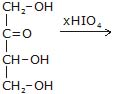x is moles of HIO4 consumed

Solution: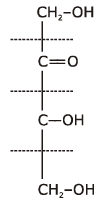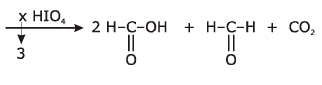QUESTION: 2

###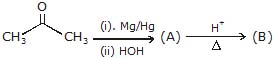Product (B) is -

Solution: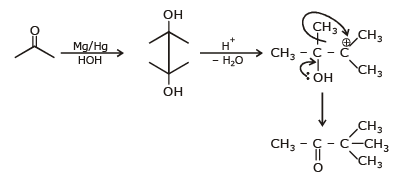QUESTION: 3

###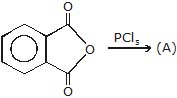; Product (A) is -

Solution: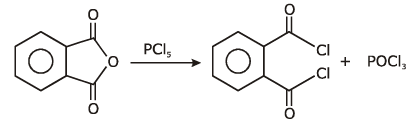QUESTION: 4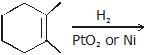Product of above reaction is obtained by

Solution: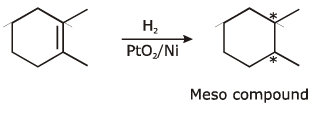QUESTION: 5

The structure of the product formed in the reaction given below is -Solution: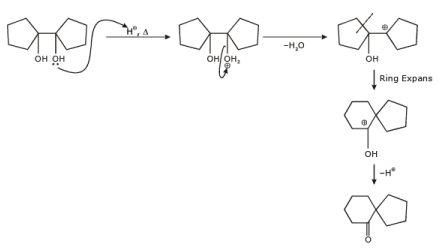QUESTION: 6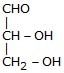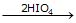, Products obtained in the above reaction are -

Solution: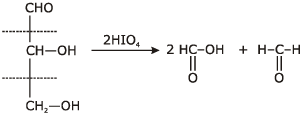QUESTION: 7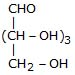+ 4HIO4 ¾→, Products obtained are

Aldo pentose

Solution: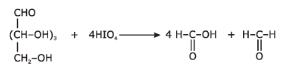QUESTION: 8

Which of the following compound gives 2HCHO, CO2, 2HCO2H when oxidisized by periodic acid

Solution: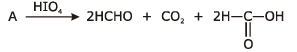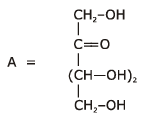QUESTION: 9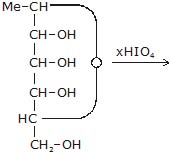,

What is the maximum value of (x) ?

Solution: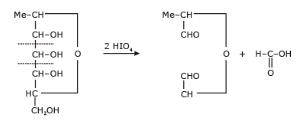QUESTION: 10

An ether is more volatile than an alcohol having the same molecular formula. This is due to

Solution:
QUESTION: 11

In the given reaction :

CH3_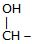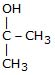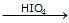(a) + (b)

(a) and (b) respectively be -

Solution: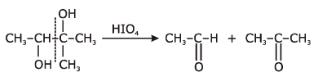QUESTION: 12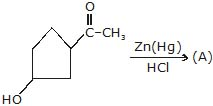;

Identify the A.

Solution: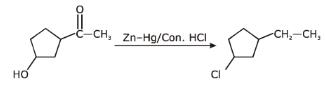QUESTION: 13

Ph–CH2–Cl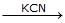(A)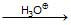(B)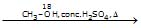(C)

product (C) is -

Solution: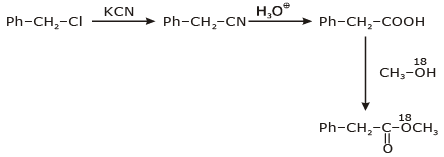QUESTION: 14

Ph–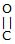–CH2–O–Et(A)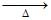(B)

Product (B) is -

Solution: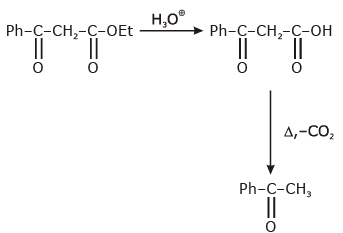QUESTION: 15

R––O–H+R1–OH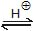R––O–R,

In above esterification reaction rate of reactin is maximum, when R1–OH is -

Solution: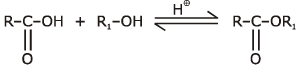Order of alcohal towards esterification
CH3–OH > 1°alcohal > 2°alcohal > 3°alchoal.

QUESTION: 16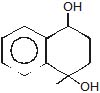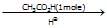(A)

product (A) is -

Solution: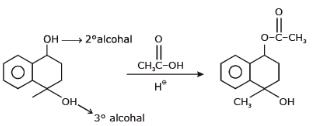QUESTION: 17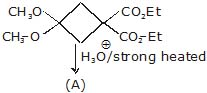;

Product (A) is -

Solution: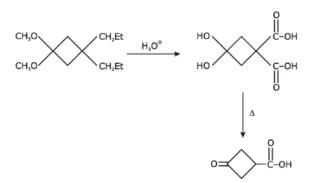QUESTION: 18

R1–OH + R – OH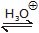R1–O–R–+H2O

In above esterification reaction rate of reaction maximum when -

Solution: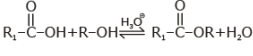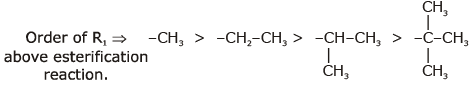QUESTION: 19

Ph–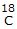–CHO18 is present in -

Solution: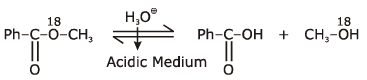QUESTION: 20

Ph––O–CH3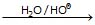Above reaction is known as -

Solution: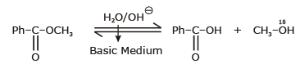Hydrolysis of ester in basic Medium is known as saponification.

QUESTION: 21

CH3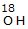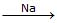(A) + B (gas)
CH3__O_CH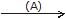(C)

Product (C) of above reaction is -

Solution: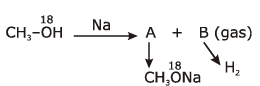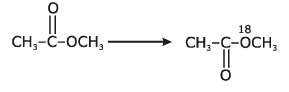QUESTION: 22

CH3–CH2–O–Et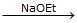(A)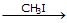(B)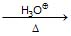(C)

Product (C) is -

Solution: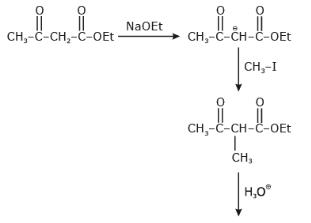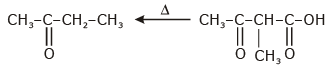QUESTION: 23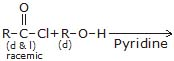Product of above reaction is -

Solution: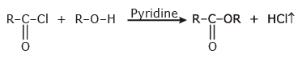HCl is formed in above reaction and react with pyridine and gives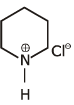QUESTION: 24

Ph––O–H+CH3–O18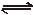(X) + H2O

Solution: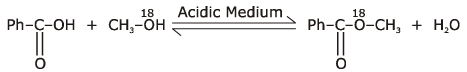This is a esterfication reaction.

QUESTION: 25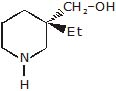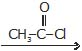(A) (major)

Solution:QUESTION: 26

Which of the following compound reduces by DIBAL-H ?

Solution:
QUESTION: 27

Which of the following compound reduces by NaBH4 ?

Solution:

NaBH4 are weak reducing agent so only acid chloride are reduced in alcohal.

QUESTION: 28

Which of the following compounds not reacts with NaOH ?

Solution: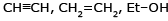are not react with NaOH.

QUESTION: 29

EtOH+CH3––O–CH3 ¾→

In above reaction molecular weight of alcohol increases by

Solution: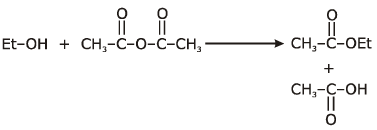in above reaction molecular weight of alcohal increase by C2H2O = 42.

QUESTION: 30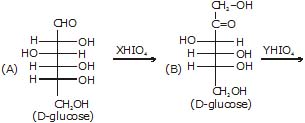x & y are moles of HIO4 consumed is above reaction.

(i) Value of x inabove reaction is -

Solution: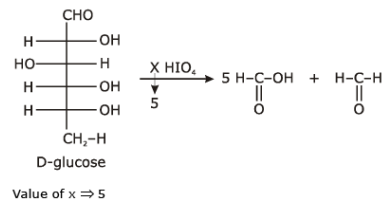QUESTION: 31x & y are moles of HIO4 consumed is above reaction.

Sum of x + y is -

Solution: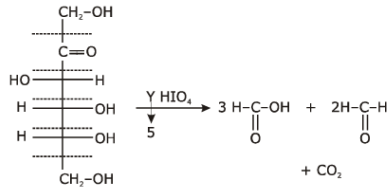QUESTION: 32x & y are moles of HIO4 consumed is above reaction.

Mole of HCHO formed in (A) is -

Solution:

Total no. of HCHO in A = 1